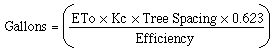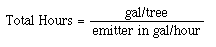College of Natural & Agricultural Sciences

# Calculating Water Applications Rates

Calculating Water Applications Rates using CIMIS

Irrigation scheduling means deciding when to irrigate and how much water to apply. The goal is to supply the plants with adequate water while minimizing loss. Some sort of irrigation scheduling is practiced by every grower, however, the bases for making decisions vary widely. The methods used to determine water need can be roughly grouped into two categories:

1. Monitoring water status in the soil or plant (example: tensiometers)
2. Estimating crop water use based on atmospheric measurements (example: CIMIS)

There are several formulas one can use to calculate water needs based on CIMIS. The formula below if fairly simple and was used for the irrigation research recently completed. It does not include a leaching factor for salty water/soil conditions, nor does it include the formula for adjusting for rainfall.

Calculating Gallons per Tree:ETo = Cumulative ETo in inches for the period in question (from CIMIS)

Kc = Crop Coefficient

Tree Spacing = Tree spacing in square feet (20 x 20 = 400 sq ft)

0.623 = conversion factor (inches to gallons)

Efficiency = Irrigation efficiency

Tree spacing note: Smaller trees that have not filled in their planted spacing should be measured on two sides (north/south and east/west) and an average for the two sides should be multiplied to give square footage of ground covered by the tree canopy. This should be used in the formula for Tree Spacing till the trees mature and fill out.

Total Hours:

To calculate the total number of hours to irrigate, use the formula below:For Further Information

Irrigation Scheduling, a guide for efficient on-farm water management. University of California Division of Agriculture and Natural Resources Publication #21454. 1989.

For a more complete discussion and a more complex formula, visit the growers section of the AvoInfo page sponsored by the California Avocado Commission. There is a set of instructions on calculating water requirements using CIMIS.